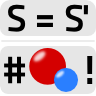## How the Gauge Invariance of the Action implies Charge ConservationUsually, gauge transformations are used to simplify certain calculations. In this problem, we want to use them to derive charge conservation from the invariance of the action $$S$$.

## Problem Statement

Let us make ourselves familiar with the implications of gauge invariance. Usually we get to know the gauge transformations as a technical trick. Nevertheless, there is much more behind it - following Noether's theorem, any transformation of the action implies a conserved current and charge as we already know from theoretical mechanics.

In electrodynamics, one example is the conservation of electric charge. Let us understand the principle in two steps:

1. Show that the electric and magnetic fields are invariant under the gauge transformations$\begin{eqnarray*} \phi\left(x^{\mu}\right)\rightarrow\phi^{\prime}\left(x^{\mu}\right)&=&\phi\left(x^{\mu}\right)-\partial_{t}\lambda\left(x^{\mu}\right)\ \text{and}\\\mathbf{A}\left(x^{\mu}\right)\rightarrow\mathbf{A}^{\prime}\left(x^{\mu}\right)&=&\mathbf{A}\left(x^{\mu}\right)+\nabla\lambda\left(x^{\mu}\right)\ , \end{eqnarray*}$where $$\lambda\left(x^{\mu}\right)$$ is an (almost) arbitrary scalar function.
2. • Calculate the change of the action $$S=\int\mathcal{L}\left(x^{\mu}\right)dVdt$$ with respect to an arbitrary gauge transformation. The condition $$S=S^{\prime}$$ results in a well known condition you may use to derive charge conservation.